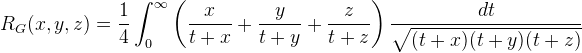Computes the Carlson integral RG.

Namespace:  Meta.Numerics.Functions
Assembly:  Meta.Numerics (in Meta.Numerics.dll) Version: 4.1.4Syntax
```public static double CarlsonG(
double x,
double y,
double z
)```

#### Parameters

x
Type: SystemDouble
The first argument, which must be non-negative.
y
Type: SystemDouble
The second argument, which must be non-negative.
z
Type: SystemDouble
The third argument, which must be non-negative.

#### Return Value

Type: Double
The value of RG(x, y, z).Exceptions
ExceptionCondition
ArgumentOutOfRangeExceptionx, y, or z is negative.Remarks

The Carlson G integral is:As can be seen from the definition, it is symmetric with respect to interchanges of any of its arguments.

The Carlson integrals can be used to express integrals of rational functions. In that sense, they are replacements for the Legendre elliptic functions.See Also